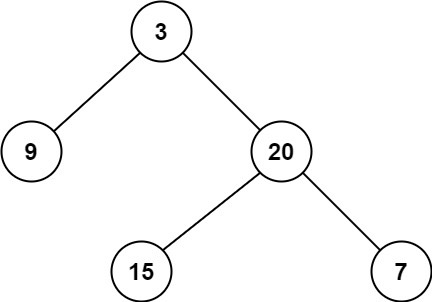# 二叉树的最小深度（ LeetCode 111 ）

## 一、题目描述输入：root = [3,9,20,null,null,15,7]



输入：root = [2,null,3,null,4,null,5,null,6]



• 树中节点数的范围在 [0, 10^5]
• -1000 <= Node.val <= 1000

## 三、参考代码

### 1、Java 代码

// 登录 AlgoMooc 官网获取更多算法图解
// https://www.algomooc.com
// 作者：程序员吴师兄
// 代码有看不懂的地方一定要私聊咨询吴师兄呀
//  二叉树的最小深度（ LeetCode 111 ）:https://leetcode-cn.com/problems/minimum-depth-of-binary-tree/
class Solution {
public int minDepth(TreeNode root) {
// 边界情况处理
if (root == null) return 0;

// 设置一个队列，用来存储二叉树中的元素

// 队列添加二叉树的根节点

// 设置 depth 用来保存输出结果
int depth = 0;

// 遍历队列，直到队列为空，说明访问了二叉树中所有的节点
while (!nodeQueue.isEmpty()) {

// 用来记录 queue 的长度，即每层节点的个数
int size = nodeQueue.size();

// 每到一层，深度就 +1
depth++;

// 使用 for 循环，将 nodeQueue 中的元素统计
for (int i = 0; i < size; i++) {

// 从 queue 中取出一个节点，此时，nodeQueue 已经抛出了这个节点
TreeNode curNode = nodeQueue.poll();

// curNode.left == null && curNode.right == null
// 说明是叶子结点
// 由于【最小深度是从根节点到最近叶子节点的最短路径上的节点数量】
// 直接返回 depth
if(curNode.left == null && curNode.right == null){
return depth;
}

// 判断当前节点的左子节点是否有值，如果有，则添加到 nodeQueue 中
if (curNode.left != null){
}

// 判断当前节点的右子节点是否有值，如果有，则添加到 nodeQueue 中
if (curNode.right != null){
}

}
}

// 返回 depth
return depth;
}
}


### 2、C++ 代码

// 登录 AlgoMooc 官网获取更多算法图解
// https://www->algomooc->com
// 作者：程序员吴师兄
// 代码有看不懂的地方一定要私聊咨询吴师兄呀
//  二叉树的最小深度（ LeetCode 111 ）:https://leetcode-cn->com/problems/minimum-depth-of-binary-tree/
class Solution {
public:
int minDepth(TreeNode* root) {
// 边界情况处理
if(root == NULL) return 0;

// 设置一个队列，用来存储二叉树中的元素

queue<TreeNode *> nodeQueue ;

// 队列添加二叉树的根节点
nodeQueue.push(root);

// 设置 depth 用来保存输出结果
int depth = 0;

// 遍历队列，直到队列为空，说明访问了二叉树中所有的节点
while (!nodeQueue.empty()) {

// 用来记录 queue 的长度，即每层节点的个数
int size =  nodeQueue.size();

// 每到一层，深度就 +1
depth++;

// 使用 for 循环，将 nodeQueue 中的元素统计
for (int i = 0 ; i < size ; i++) {

// 从 queue 中取出一个节点，此时，nodeQueue 已经抛出了这个节点
TreeNode *curNode = nodeQueue.front();

nodeQueue.pop();

// curNode->left == NULL && curNode->right == NULL
// 说明是叶子结点
// 由于【最小深度是从根节点到最近叶子节点的最短路径上的节点数量】
// 直接返回 depth
if(curNode->left == NULL && curNode->right == NULL){
return depth;
}

// 判断当前节点的左子节点是否有值，如果有，则添加到 nodeQueue 中
if (curNode->left != NULL){
nodeQueue.push(curNode->left);
}

// 判断当前节点的右子节点是否有值，如果有，则添加到 nodeQueue 中
if (curNode->right != NULL){
nodeQueue.push(curNode->right);
}

}
}

// 返回 depth
return depth;
}
};


### 3、Python 代码

# 登录 AlgoMooc 官网获取更多算法图解
# https://www.algomooc.com
# 作者：程序员吴师兄
# 代码有看不懂的地方一定要私聊咨询吴师兄呀
#  二叉树的最小深度（ LeetCode 111 ）:https://leetcode-cn.com/problems/minimum-depth-of-binary-tree/
class Solution:
def minDepth(self, root: TreeNode) -> int:
# 边界情况处理
if root == None :
return 0

# 设置一个队列，用来存储二叉树中的元素
nodeQueue = deque()

# 队列添加二叉树的根节点
nodeQueue.append(root)

# 设置 depth 用来保存输出结果
depth = 0

# 遍历队列，直到队列为空，说明访问了二叉树中所有的节点
while nodeQueue :

# 用来记录 queue 的长度，即每层节点的个数
size =  len(nodeQueue)

# 每到一层，深度就 +1
depth += 1

# 使用 for 循环，将 nodeQueue 中的元素统计
for i in range(size):

# 从 queue 中取出一个节点，此时，nodeQueue 已经抛出了这个节点
curNode = nodeQueue.popleft()

# curNode.left == None && curNode.right == None
# 说明是叶子结点
# 由于【最小深度是从根节点到最近叶子节点的最短路径上的节点数量】
# 直接返回 depth
if curNode.left == None and curNode.right == None :
return depth

# 判断当前节点的左子节点是否有值，如果有，则添加到 nodeQueue 中
if curNode.left :
nodeQueue.append(curNode.left)

# 判断当前节点的右子节点是否有值，如果有，则添加到 nodeQueue 中
if curNode.right :
nodeQueue.append(curNode.right)

# 返回 depth
return depth